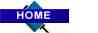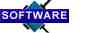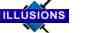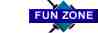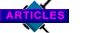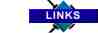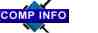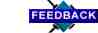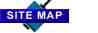Color Math

Introducing a color-related concept that is highly innovative and exciting yet dangerously controversial and for the moment, unscientifically proven. It's called Color Math.

Using Color Math, we can calculate how to mix colors mathematically. This article explains the basic principles of color math and runs through some simple scenarios. The color system we will be using is subtractive color (such as mixing paint).

 Download article in MS Word format.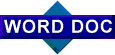to go to order page

Other Articles:
Color Poster

Color Basics
Color Mixing
Color Models
The Color of Music
Prismology
Color Math
Color Glossary
Color Theory
Color Techniques

The Color Math Concept
by Ken Davies

The human eye is able to decipher patterns of light according to the primary colors of the additive system: red, green and blue (RGB). However, it is the subtractive system’s primaries: cyan, magenta and yellow (CMY) that best lend themselves to understanding the COLORCUBE and the concept of Color Math. This chapter unveils the inner workings of the COLORCUBE Model and the tools that are required for navigation in and about it.

Within any given subtractive color space, as defined by the three primaries CMY, the use of Color Math will allow us to map the relationships of all the colors encompassed by that cubic area. Bridging the additive and subtractive systems of color, mixing colors, selecting color complements and converting color media equivalents all become matters of mathematics rather than that of guess work or compromise.

Following a brief introduction to basic logic and scientific fundamentals, as they are relevant to color, we will expand upon the Color Math concept. By the conclusion of this chapter, we will be able to apply Color Math to a variety of tasks such as dissecting the COLORCUBE, dispelling critical aspects of traditional color theory, mixing colors, determining color complements and charting print-to-paint media conversions.

Principles of Color Math

The similarity to commonly applied basics in mathematics and algebra will make these initial color principles appear somewhat elementary. However, their value will become apparent as the problems that we will encounter become increasingly complex.

The diagram below illustrates the principle of symmetry that states that the order in which colors are added to one another does not alter the outcome.

 Figure 1. Color A + Color B = Color B + Color A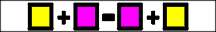The addition (or subtraction) of two or more colors will likely cause a visible change in hue but we must also pay particular attention to the cumulative volume of the operation. For example, mixing one measurable unit of color with another yields twice the volume of the resulting color. The following diagram highlights this change in quantity using simple, like colors.

 Figure 2(a). 1 part Color A + 1 part Color A = 2 parts Color AAlso, this principle of cumulative volume applies to those operations involving varying colors as well.

 Figure 2(b). 1 Color A + 1 Color B = 2 Color C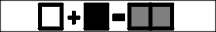As easy as these fundamentals are to grasp, the visual aids accompanying the upcoming problems shown may be counter-intuitive. The derivations of the Color Math "building blocks" presented in the next section will be the first step in making them fully comprehensible.

Primary Colors

We have stated that all of the colors captured within a color space are functions of the primaries cyan, magenta and yellow. The vertices or corner points on a COLORCUBE are these three pure hues (labeled A, B and C below), along with their various combinations. Together, these eight colors represent the key elements used in all Color Math calculations.

 Figure 3. Primary colors in the subtractive systemAlthough considered primary in the additive system of color, red, green and blue are the secondary colors in the subtractive system. By combining cyan with magenta, cyan with yellow and magenta with yellow, we are able to make blue, green and red respectively. As long as each primary ingredient is at full saturation and in equivalent quantities, the following operations hold true.

 Figure 4(a). 1 Cyan + 1 Magenta = 2 BlueFigure 4(b). 1 Cyan + 1 Yellow = 2 Green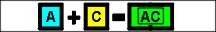Figure 4(c). 1 Magenta + 1 Yellow = 2 RedWhen all three primaries are mixed together in equal proportion, the resulting color is black. (editor’s note: this color mixture is mainly theoretical as we have yet to encounter perfect subtractive primaries that make a perfect black when mixed.)

 Figure 5. 1 Cyan + 1 Magenta + 1 Yellow = 3 BlackBlack is also achieved when certain combinations of the primary and secondary elements are mixed. We recall from figure 4 (c), that red is made up of equal parts magenta and yellow. Therefore, we can add cyan to red in order to form black by virtue of the equation below:

 Figure 6. Color A + SUM Colors BC = Color ABC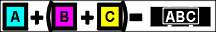And so,

 Figure 7(a). 1 part Cyan + 2 parts Red = 3 parts Black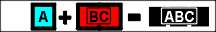Because each secondary color is merely a combination of two primaries, black is the result in each of the following mixtures. Please note the unequal quantities of the inputs and the constant amount of the sum.

 Figure 7(b). 1 Magenta + 2 Green = 3 Black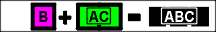Figure 7(c). 1 Yellow + 2 Blue = 3 BlackThe operations shown above may beg the question: what happens when the secondary colors are added together in equal quantities, as in the diagram below?

 Figure 8. Red + Green + Blue?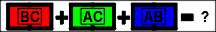Answering this question correctly requires a break down of each of the inputs into its’ constituent CMY elements in order to investigate the exact quantities being mixed. In order to simplify this task, we will take single units of red, green and blue and discover that they yield the following:

 Figure 9. Adding Red + Green + Blue (in terms of CMY)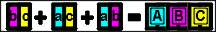Upon analyzing the CMY proportions in the sum, we recognize the color as black! This solution may strike you as odd. After all, if equal quantities of primary colors cyan, magenta and yellow make black, how can the same amounts of secondary colors red, green and blue possibly produce an identical result? Can the following diagram really be true?

 Figure 10. 1 Red + 1 Green + 1 Blue = 3 Black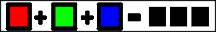The answer is "yes" because we have learned that, by definition; black is CMY in equal amounts at full saturation. (If the saturation or purity of the primary hues is less than full, some form of gray will emerge from the mixture. The value of the gray will depend on the amount of base color present.) So, in deconstructing red, green and blue into their respective CMY elements, we discover that these three colors cumulatively do indeed carry the correct CMY proportions to create black. Color Math will be used later in the chapter to prove the validity of this conclusion.

White as "Base" Color

In the parentheses above, we alluded briefly to the concept of the base color. This is the color that is perceived when no primary hues are present. For instance, in color media that utilize the additive system of color, black is considered to be the base color. That is, the eye responds to the absence of red, green and blue (primaries in the additive system) and its perception is the color black. The opposite occurs in the subtractive system where zero values of cyan, magenta and yellow result in the perception of white.

While the use of a non-white, non-black base is possible; the result is a truncated colorspace and a somewhat skewed COLORCUBE. Such is the case when looking through tinted eyewear or offset printing onto colored paper. Thus, in order to maximize the set of visible colors within our CMY color space, white will be the assumed base color.

To this point in our discussion, all of the colors have been fully saturated containing no white or base color. We now attempt to understand the impact of the base color by infusing white base with increasing amounts of primary hues and observe the changes to the resulting color.

Our analysis begins with total white base to which we add rising increments of yellow primary. As long as the overall volume of each color sample is held constant, the progression from 100% white leads to a fully saturated yellow. As the horizontal bars in the top left diagram show, the reduction of base color, white gives way to an increase of non-base content. The changes in the "base-to-primary" ratio results in graduated versions of a mixed color (known as tints due to the presence of white) as shown in the color circles. The upper right corner of the diagram below maps the same procedure using magenta primary.

 Figure 11. Adding primaries to a white base.Let us now conduct a similar operation with two primary colors adding them both to the base color, white. We should recognize that the combination of equal parts yellow and magenta creates red but as illustrated above in the bottom left box, this mixture does not affect the proportion of the white base. The base color maintains its percentage value of 100, 75, 50, 25 and 0% of the total color respectively, regardless of the amounts of multiple primaries which make up the remainder.

The same effect takes place when all three primaries are added to decreasing amounts of white base. As demonstrated in the bottom right corner above, grays and black are the result of mixing cyan, magenta and yellow with a white base. It is important to remember from these exercises that the CMY primary mixture occupies only the portion of the color that is non-base.

Constructing the COLORCUBE

Returning momentarily to the progressions of magenta and yellow tints, we in fact derived two axes of the COLORCUBE. The plane of color, constructed in the figure 12, is what bridges the color space that exists between those two axes. Using a similar strategy to that exhibited earlier, we start with 100% white and incrementally reduce the amount of base color while increasing the primary content. The non-base portions of each color shown in the diagram are mixtures containing magenta and/or yellow. Horizontal bars once again capture the systematic increases in these mixtures within the fixed overall volume of color.

 Figure 12. White-Yellow/Magenta plane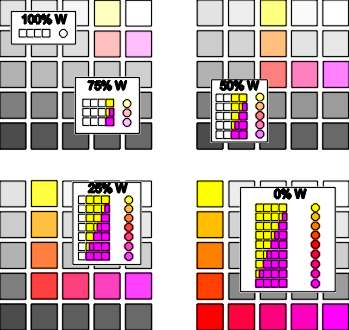It should seem obvious that moving away from the white square horizontally implies a larger presence of yellow and likewise, a vertical movement downward results in the addition of magenta. What may not be so obvious is that these increases in primaries affect only the portion of color that is non-base. For instance, although magenta and yellow are maximized at a certain point in the "25% base color" diagram, they only contribute 37.5% each to the whole color. (In case this is unclear, the "certain point" that is being referred to is in the bottom left diagram, located in the middle of the color group and is the corner of the colored squares).

The next diagram depicts the unified yellow-magenta plane previously constructed. The horizontal bars of color have been attached to their corresponding color to illustrate the proportions of base and non-base content in each. These "Color Bars" also make the pattern that exists between the relative quantities of the primaries themselves visually apparent.

 Figure 13. Unified Yellow-Magenta plane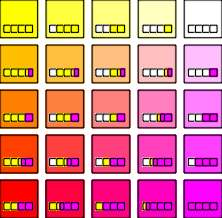We will continue to attach these Color Bars to color samples in order to assist our understanding of how the COLORCUBE is built. They are graphic and mathematical representations of the ingredients present in each color and are standardized to a constant length for the purposes of measurement and comparison.

The next phase of COLORCUBE construction involves the addition of the remaining primary, in this case, cyan. The above plane was theoretically conceptualized by taking the white-yellow axis and incrementally increasing magenta content while adjusting base content to maintain its proportion. This resulted in multiple axes containing various combinations of the white and primary colors.

The incremental addition of cyan to this entire magenta-yellow plane will yield similar results in the form of multiple planes of color. This third dimension of color, as in the diagram below, is organized in terms of base color percentage (exactly as above) and each plane is separated according to cyan content. The Color Bars once again fix the overall volume of each color to a single value and allow us to see the actual color that each formula represents.

 Figure 14. Increasing Cyan content incrementallyApplying Color Math

Having completed our derivation of an entire COLORCUBE, we revisit the color theory basics from the beginning of the chapter and attempt to prove these operations using Color Math. In doing so we will introduce tools and techniques that will assist our understanding of CMY addition and subtraction as well as create a visual grounding to Color Math procedures.

Color Bars

 Horizontal bars of color were used extensively during the construction of the COLORCUBE to graphically depict the base and non-base content of each color they represented. They were extremely useful in allowing us to detect and map the patterns that exist within a color space. In this section these diagrams become accessories to the addition of color and precursors to actual Color Math. Figure 15.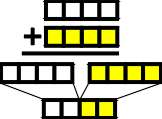Remembering that each Color Bar is standardized to a single set length, we are able to add these fixed amounts together in order to determine the configuration of their sum.

For example, a given quantity of 100% white plus an equal amount of 100% primary yellow would yield a color that is half base, half non-base with a 50% yellow hue.

Figure 15 demonstrates this graphically. It shows that once the input colors are combined and the mixture is reset to the size standard of the original Color Bar(s), the resulting color is one that contains equal parts white base and yellow primary.

 Figure 16. The diagram to the left is another situation that involves base white. However, this example shows the second input to be a color containing unequal multiple primaries. When these Color Bars are added together we can see that the sum contains four parts base white to four parts non-base primary, which is made up of three parts magenta and one part yellow. Once this result is subsequently resized to the standardized length, we learn that the four white squares are proportionately equal to half of the resulting color. Likewise, the primaries magenta and yellow occupy 1.5 and .5 of the remaining units, respectively.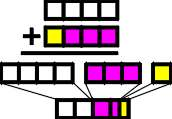When adding colors using Color Bars, the presence of three primaries adds complexity to the equation but the procedure for solving it is the same.

 Figure 17 shows the summarization of three mixed colors. Each of the ingredients are gathered and grouped according their CMYW category. After this sorting process, these input values are sized down to the normal Color Bar in order to discover the formula of the resulting color. Grasping the need to calibrate all color combinations to a fixed amount is important because this normalization process allows us to estimate the colors with which we are dealing using the cube diagrams in the previous section. Having become familiar with the patterns inherent to the COLORCUBE, we can navigate within that color space using the Color Bars as guides. Figure 17.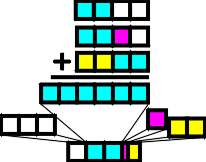Figure 18. Let us take for our next equation the sums of the last two operations and use them as inputs. After adding the Color Bars together, we can sort and recalibrate the sum to a fixed amount with all of the constituent elements intact. It should be clear by now that the usefulness of this "Color Bar" addition is severely compromised by the increasing complexity of the operations and a general inability to distinguish minute proportions with any degree of precision. Alleviating this requires a Color Math process that relies strictly on the objectivity of percentage values and quantities of color rather than that of a guessing science.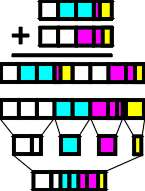Color Boxes

A method that addresses our need for exact numbers while retaining the benefits granted us by the Color Bars must begin with the information on hand being organized in a practical manner. The tables introduced here place the CMY formula of a color in a usable format that allows any color to be added and/or subtracted. It also takes into consideration the volume of color as well.

Suppose we have three units of color gold (C20 M40 Y60) which is one of our inputs in a Color Math operation. Our first step is to determine its percentage base content by subtracting the CMY quantity that is greatest from 100. In this instance, yellow is most dominant with a value of 60. Consequently, this color contains 40% base white.

The remaining 60% consists of primary colors cyan, magenta and yellow in relative proportion to the color’s CMY formula. Thus, we divide each of the primary quantities (20, 40, and 60) by the sum of those primaries (120) and multiply each by 60%. This yields adjusted weights of 10, 20 and 30% for CMY respectively. We find that this shade of gold is 10% cyan, 20% magenta, 30% yellow and 40% white. The table below summarizes this information as well as provides the volume calculation.

 Figure 19. Table for CMY breakdown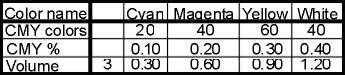The next diagram displays the above information in a graphical format that actually maps the relative strength of each of the CMY element and places the overall proportion of the base color in the forefront. This Color Box format adds a visual dimension to the CMY formula and offers insight to the color itself.

 Figure 20. Color Box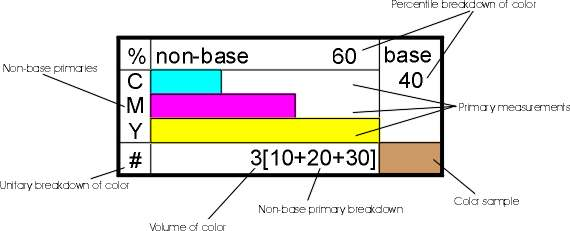This Color Math documentation also makes use of tables and diagrams that isolate the current operation. These are fairly self-explanatory in that they summarize the actual quantities and volumes of color that are being added or subtracted in columnar form. In figure 21, "A" and "B" represent the input colors and "C" symbolizes the resultant color.

 Figure 21. Table of operation and accompanying graphic.Introduction Complete

At this point, the basics of Color Math have been fully explained. We pause here to welcome comments from you that either accept or reject the theories explored here. Please contact Spittin’ Image Software for more information on Color Math and look for future developments on this fascinating subject on this website.